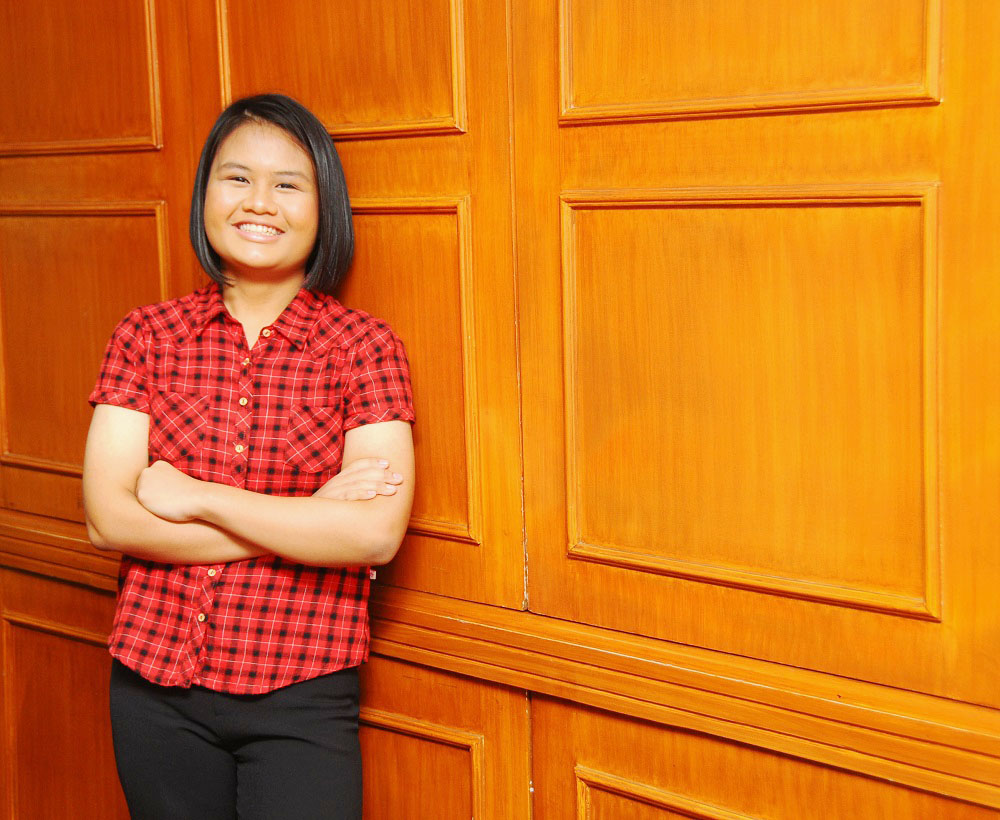# Ninth Grader Aces Math Certification ContestA Grade 9 student from Southville International School and Colleges (SISC) passed the Suken Certification Test administered by the Mathematics Certification Institute of Japan. Gwyneth Margaux Tangog of Gr. 9 – Compassion passed the 2nd Kyu level which is meant for Grade 11 students. The Suken exam has 12 certification levels called Kyus with the 1st Kyu for college graduates being the highest.

The test was divided into two parts: the “Section 1: Calculation Test” for measuring calculation skills, and the “Section 2: Application Test” for measuring applied mathematical skills. It covers topics on fractional expressions, higher-degree equations, various functions (exponential functions, logarithmic functions, trigonometric functions, higher-degree functions), locus and area, differential coefficients and derivative functions, indefinite and definite integrals, vectors, complex numbers, probability distribution, and statistical inference, etc. Tangog got more than 70% of section 1 and 60% of section 2 correct to earn the internationally recognized certification